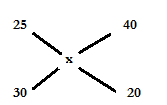# SSC CGL 2018 Practice Test Papers | Quantitative Aptitude (Day-8)

Dear Aspirants, Here we have given the Important SSC CGL Exam 2018 Practice Test Papers. Candidates those who are preparing for SSC CGL 2018 can practice these questions to get more confidence to Crack SSC CGL 2018 Examination.

[WpProQuiz 2737]

Click “Start Quiz” to attend these Questions and view Explanation

1. The sum of interior angles of a polygon of n sides is
1. (2n-4)x180
2. (2n+4)x180
3. (2n-4)x90
4. (2n-4)x90
1. If(x+1/x) = 10/3, then what is the value of x2+1/x3
1. 245/27
2. 244/9
3. 244/27
4. 243/9
1. Kiran travels 30 minutes at the speed of 25 km/hr and travels 20 minutes at the speed of 40 km/hr. Find his average speed in km/hr?
1. 33
2. 26
3. 31
4. 29
1. Two balls are drawn at random from a basket having 11 red, 7 white and 4 blue balls. What will be the probability of getting 2 blue balls?
1. 2/68
2. 2/95
3. 3/89
4. 2/77
1. Find the cost of fencing 0.5 meter boundary around a rectangular field of perimeter 156 m at the rate of 8 per square meter.
1. 632
2. 624
3. 543
4. 512
1. What is the cost of Laptop if laptop is 12000 more than the cost of tab and the tab is 2 times the cost of a mobile which costs 13000.
1. 40000
2. 36000
3. 38000
4. 42000
1. What is the principal if the sum doubles itself in 4 years at the rate of interest 25%?
1. 46000
2. 60000
3. 56000
4. cannot be determined
1. What is the smaller angle of the parallelogram if larger angle is 38o more than smaller angle?
1. 65
2. 60
3. 71
4. 80
1. 2835/25 + 0.6×5 −12.4 =?
1. 87
2. 89
3. 100
4. 104
1. 250% of 35/150 of 750=?
1. 440
2. 437.5
3. 465.5
4. 423

The sum of interior angles of a polygon of n sides is (2n-4)x90

X+1/x=10/3

X2+1=10x/3

X2-10x/3+1=0

X=3

Substitute x=3 in x2+1/x3

=32+1/33=244/9(x-25)/(40-x)=30/20

X=31

Average speed=31 km/hr

Probability of getting 2 blue balls= 4C2/22C2=(4×3/2×1)/(22×21/2×1)=2/77

Cost of laptop=12000+ cost of tab

Cost of tab= 2x cost of mobile=2×13000=26000

Cost of laptop=12000+26000=38000

Let the smaller angle be x and the larger angle be x+38 respectively.

By property of parallelogram,

X+x+38=180

X=71